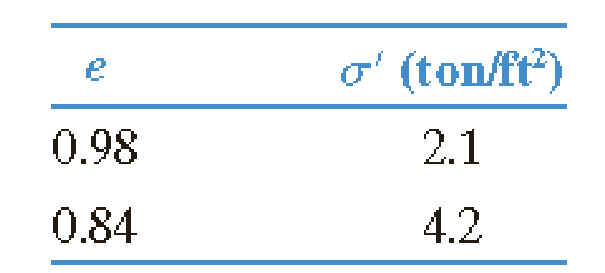Chapter 11, Problem 11.16PPrinciples of Geotechnical Enginee...

9th Edition
Braja M. Das + 1 other
ISBN: 9781305970939

Solutions

Chapter
SectionPrinciples of Geotechnical Enginee...

9th Edition
Braja M. Das + 1 other
ISBN: 9781305970939
Textbook Problem

For the consolidation test data given in Problem 11.12, a. Determine the coefficient of volume compressibility for the pressure range stated. b. If cv = 2.05 × 10–3 in.2/sec, determine k in ft/sec corresponding to the average void ratio within the pressure range. 11.12. An undisturbed clay has a preconsolidation pressure of 1.9 ton/ft2 at a void ratio of 1.1. Laboratory consolidation test yields the following data:a. Determine the void ratio that corresponds to a pressure 6 ton/ft2. b. What would be the void ratio at a pressure of 1.5 ton/ft2? Given: C s = 1 5 C c .

(a)

To determine

Calculate the coefficient of volume compressibility for the pressure range.

Explanation

Given information:

The preconsolidation pressure (σc) is 1.9ton/ft2.

The void ratio of clay (e) is 1.1.

The void ratio (e1) is 0.98.

The void ratio (e2) is 0.84.

The pressure (σ1) is 2.1ton/ft2.

The pressure (σ2) is 4.2ton/ft2.

Calculation:

Calculate the change in void ratio (Δe) as shown below.

Δe=e1e2

Substitute 0.98 for e1 and 0.84 for e2.

Δe=0.980.84=0.14

Calculate the change in pressure (Δσ) as shown below.

Δσ=σ2σ1

Substitute 2.1ton/ft2 for σ1 and 4.2ton/ft2 for σ2.

Δσ=4.22.1=2.1ton/ft2

Calculate the average void ratio (eav) as shown below

(b)

To determine

Calculate the hydraulic conductivity in ft/sec within the pressure range.

Still sussing out bartleby?

Check out a sample textbook solution.

See a sample solution

The Solution to Your Study Problems

Bartleby provides explanations to thousands of textbook problems written by our experts, many with advanced degrees!

Get Started

Give examples of the important role of volume in engineering analysis and design.

Engineering Fundamentals: An Introduction to Engineering (MindTap Course List)

What type of axle housing resembles a banjo?

Automotive Technology: A Systems Approach (MindTap Course List)

Explain how a power supply converts AC current into DC current.

Enhanced Discovering Computers 2017 (Shelly Cashman Series) (MindTap Course List)

Name metal cutting machines used in the welding shop and their advantages.

Welding: Principles and Applications (MindTap Course List)